# ML Aggarwal Solutions for Class 8 Maths Chapter 8 Simple and Compound Interest

ML Aggarwal Solutions for Class 8 Maths Chapter 8 Simple and Compound Interest has problems prepared by experienced teachers at BYJU’S. Mathematics is one such subject, where students score high marks with ease, practicing on a regular basis. These solutions help them to improve conceptual knowledge and also assist them to ace the exam. For better understanding of the concepts, students can make use of ML Aggarwal Solutions for Class 8 Maths Chapter 8 Simple and Compound Interest PDF, from the links which are mentioned below.

Chapter 8 discusses problems on finding the rate of interest, principal amount and the time period, as per the current ICSE syllabus. Following ML Aggarwal Solutions, students can cross check their answers, while solving textbook questions and also clear their doubts instantly. It also enhances their speed of solving problems, which is essential for better performance in academics.

## ML Aggarwal Solutions for Class 8 Maths Chapter 8 Simple and Compound Interest Download PDF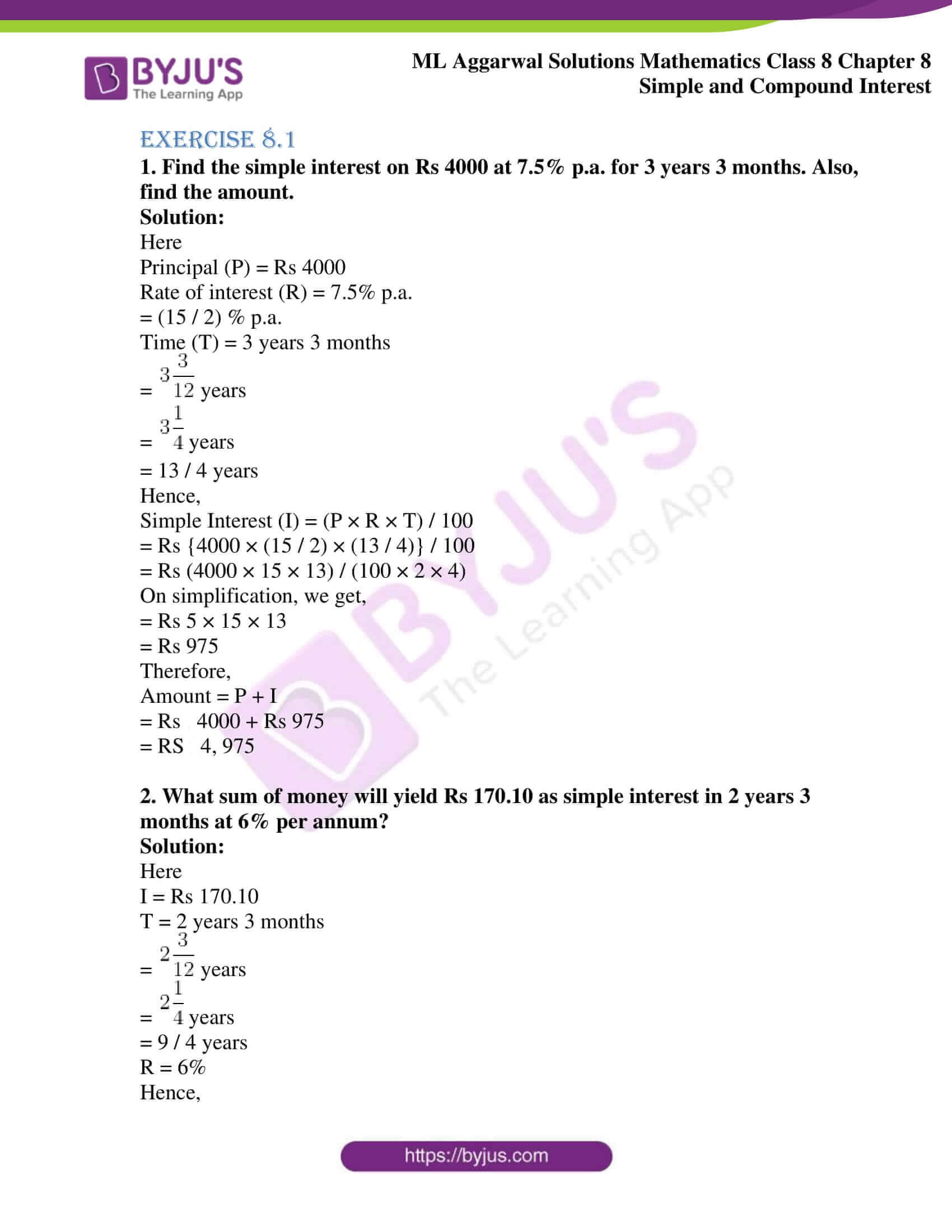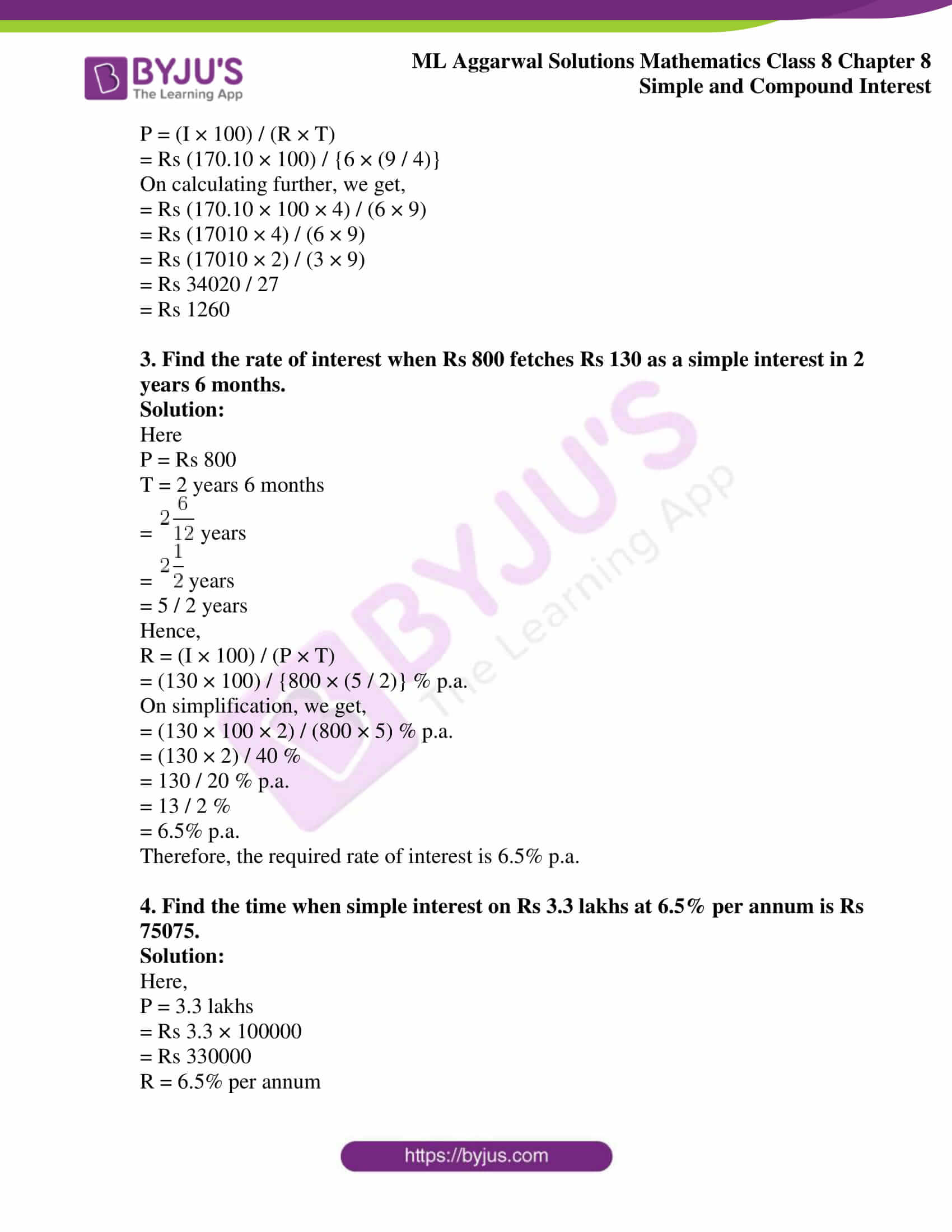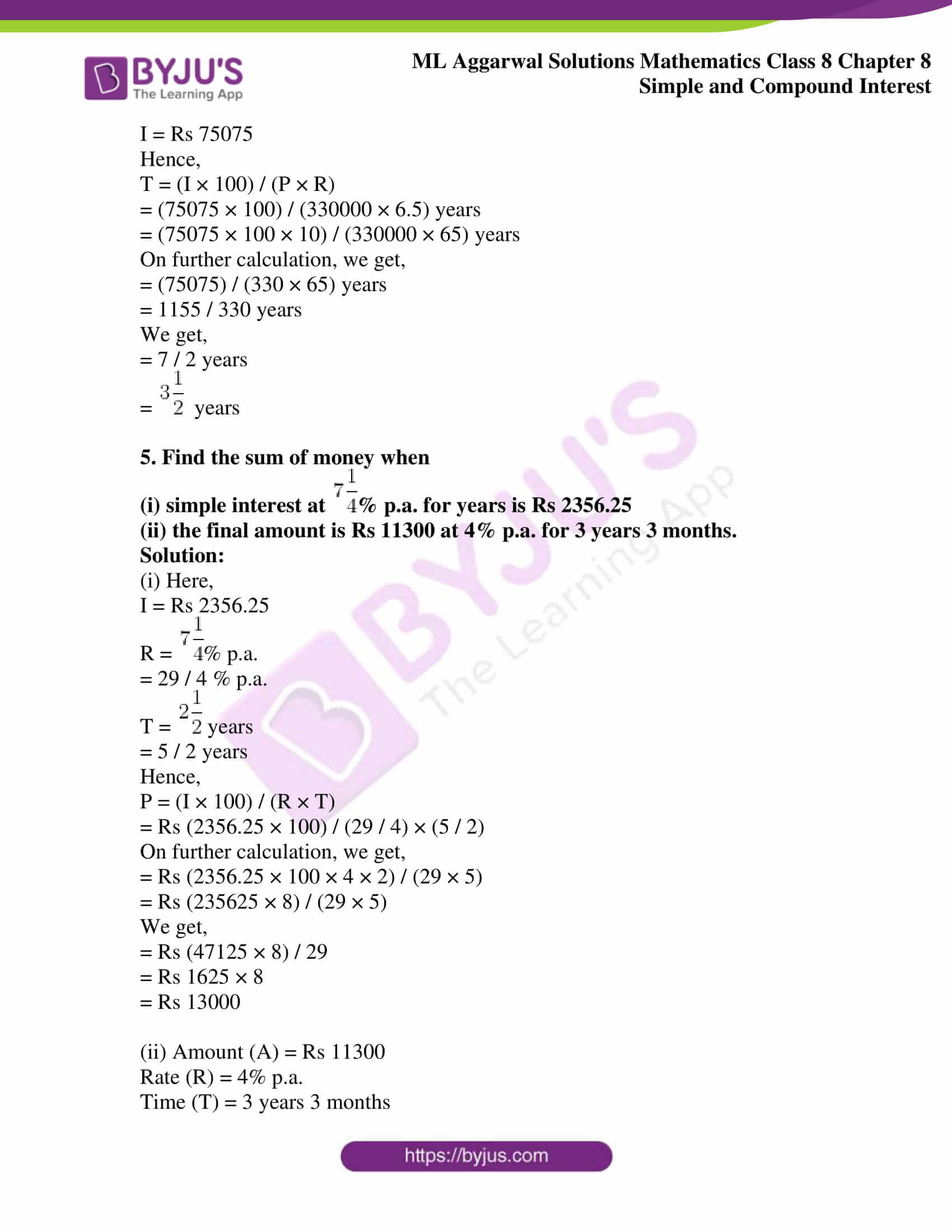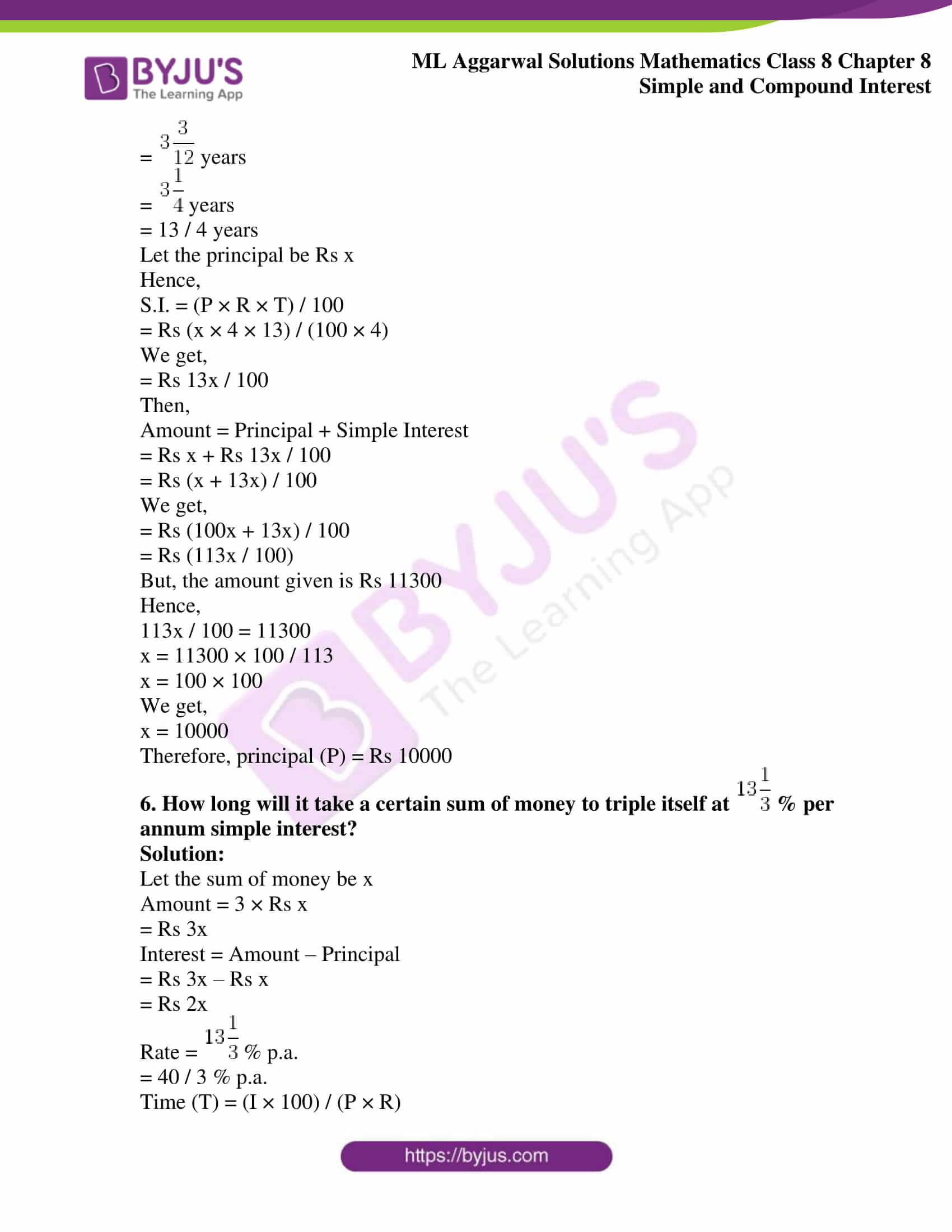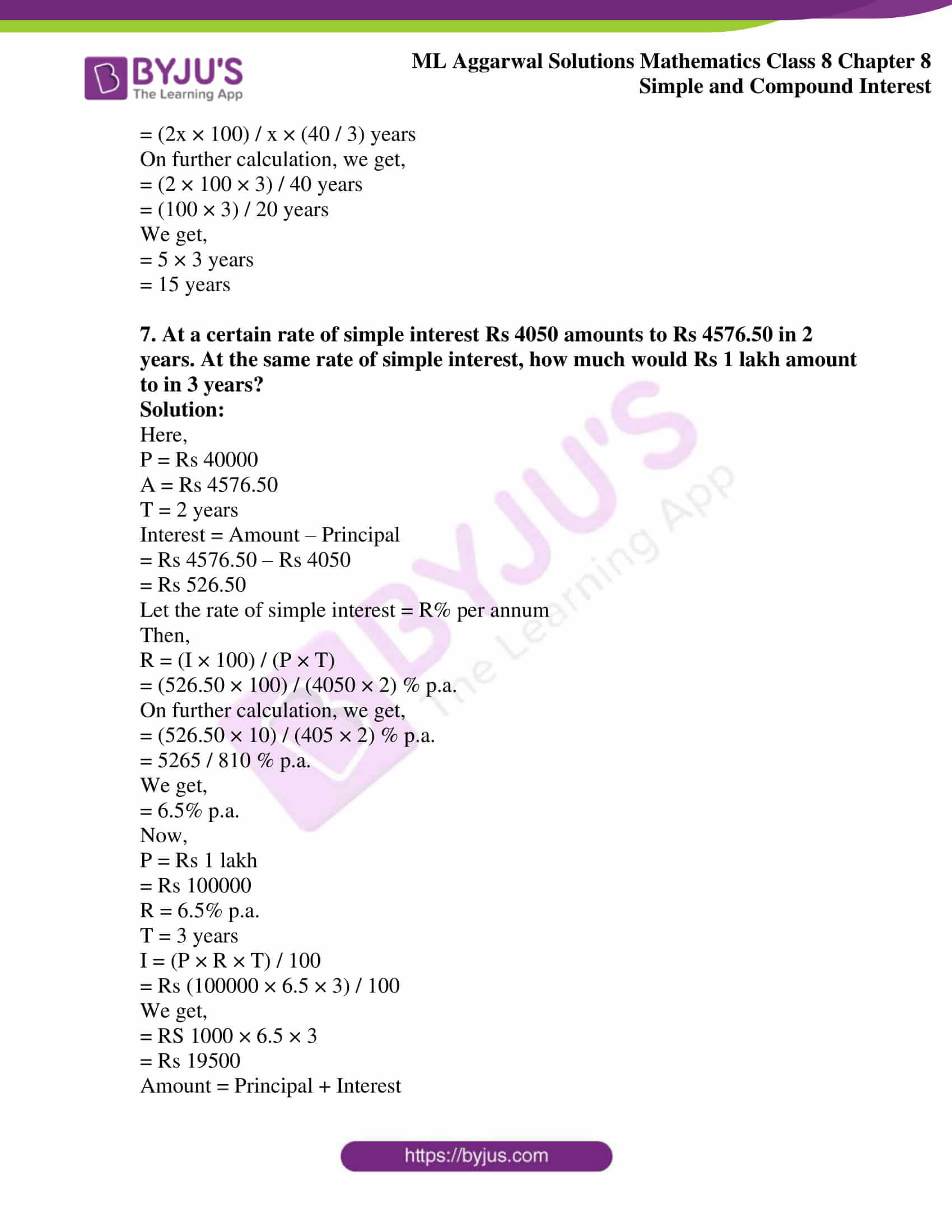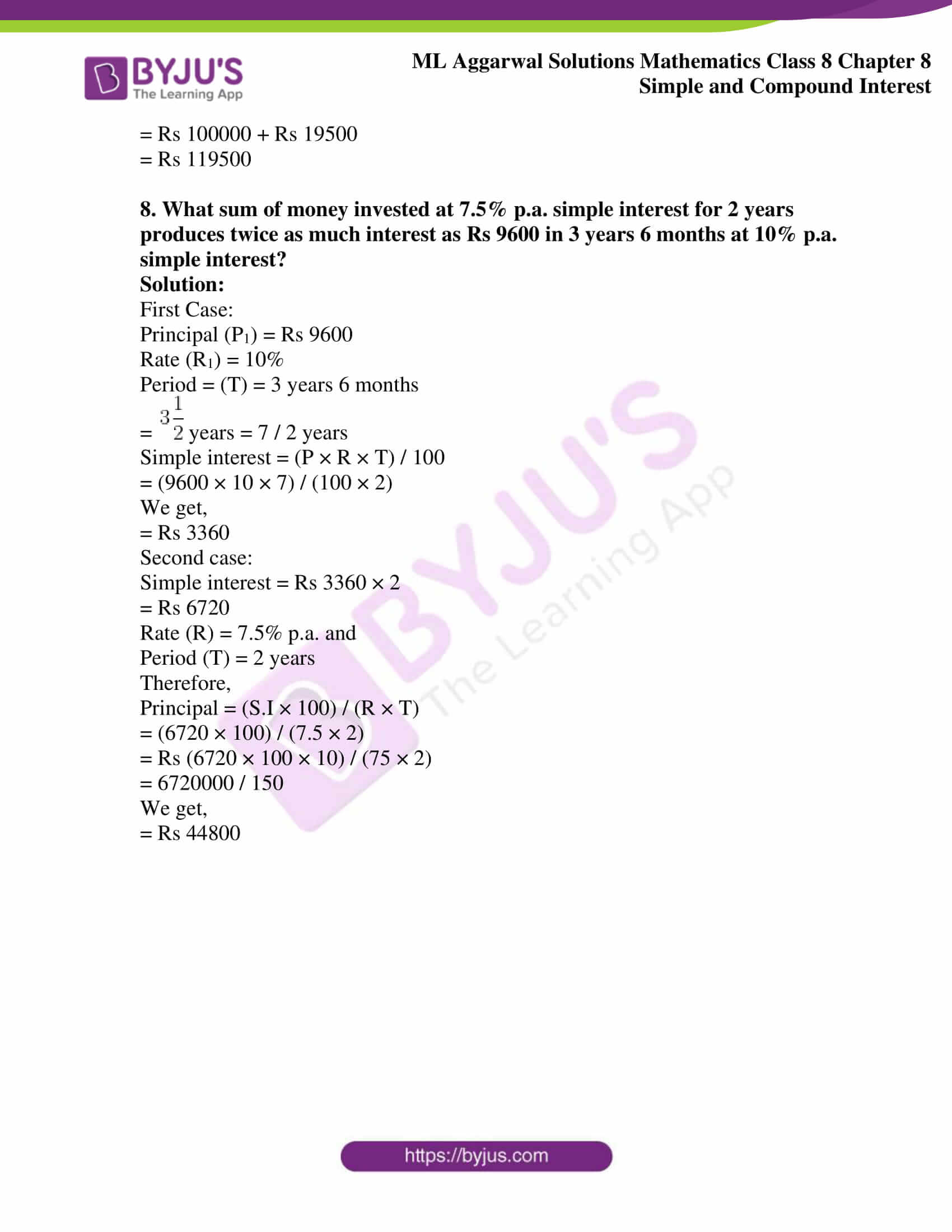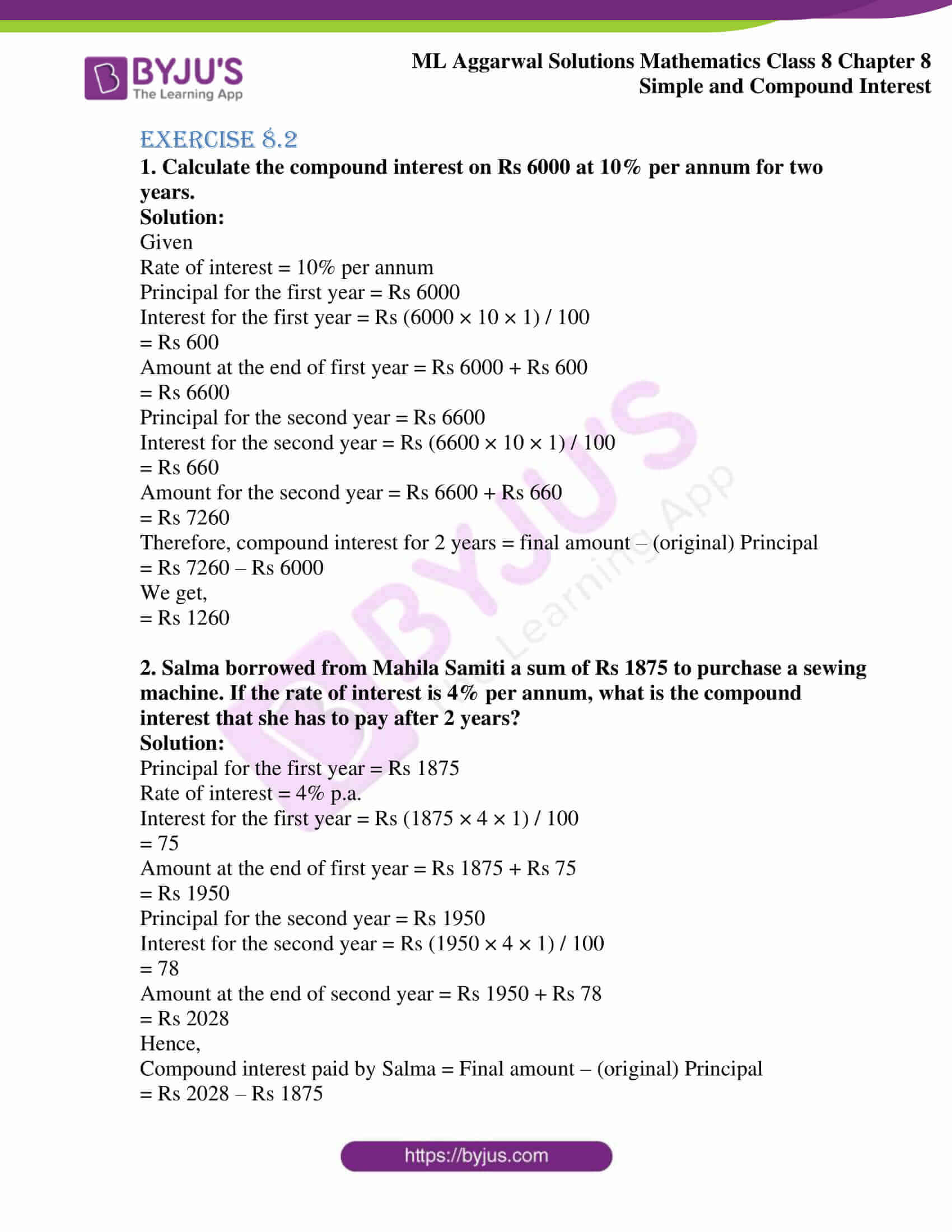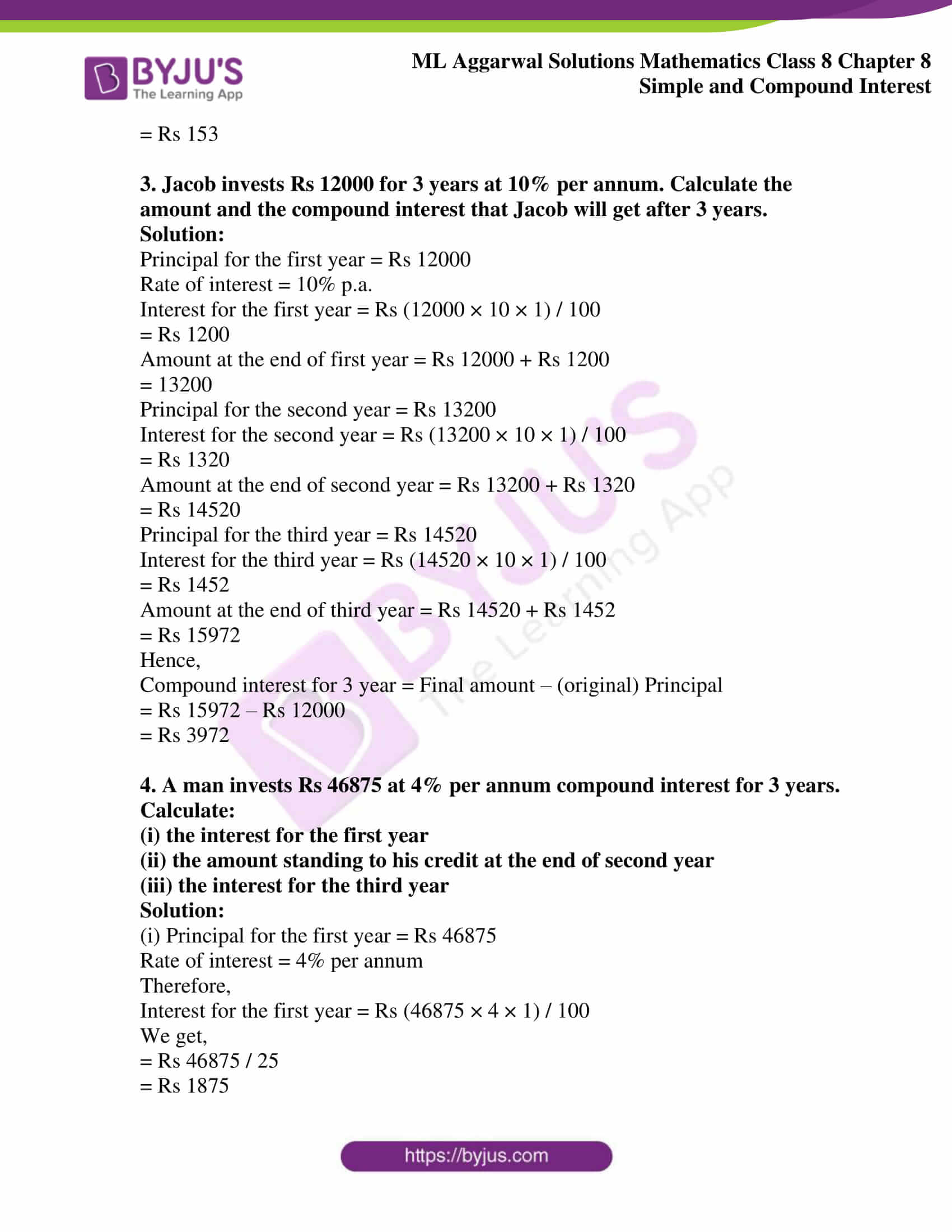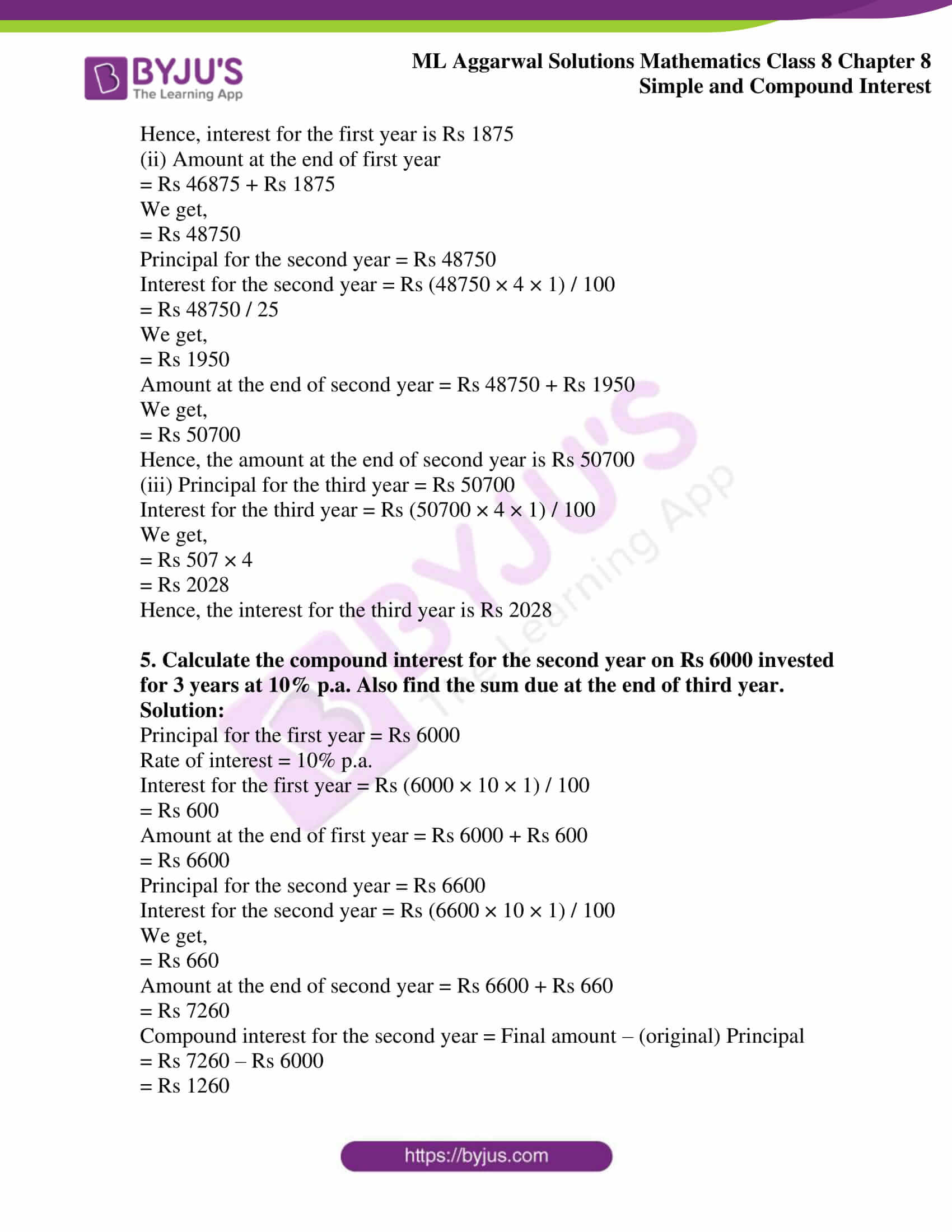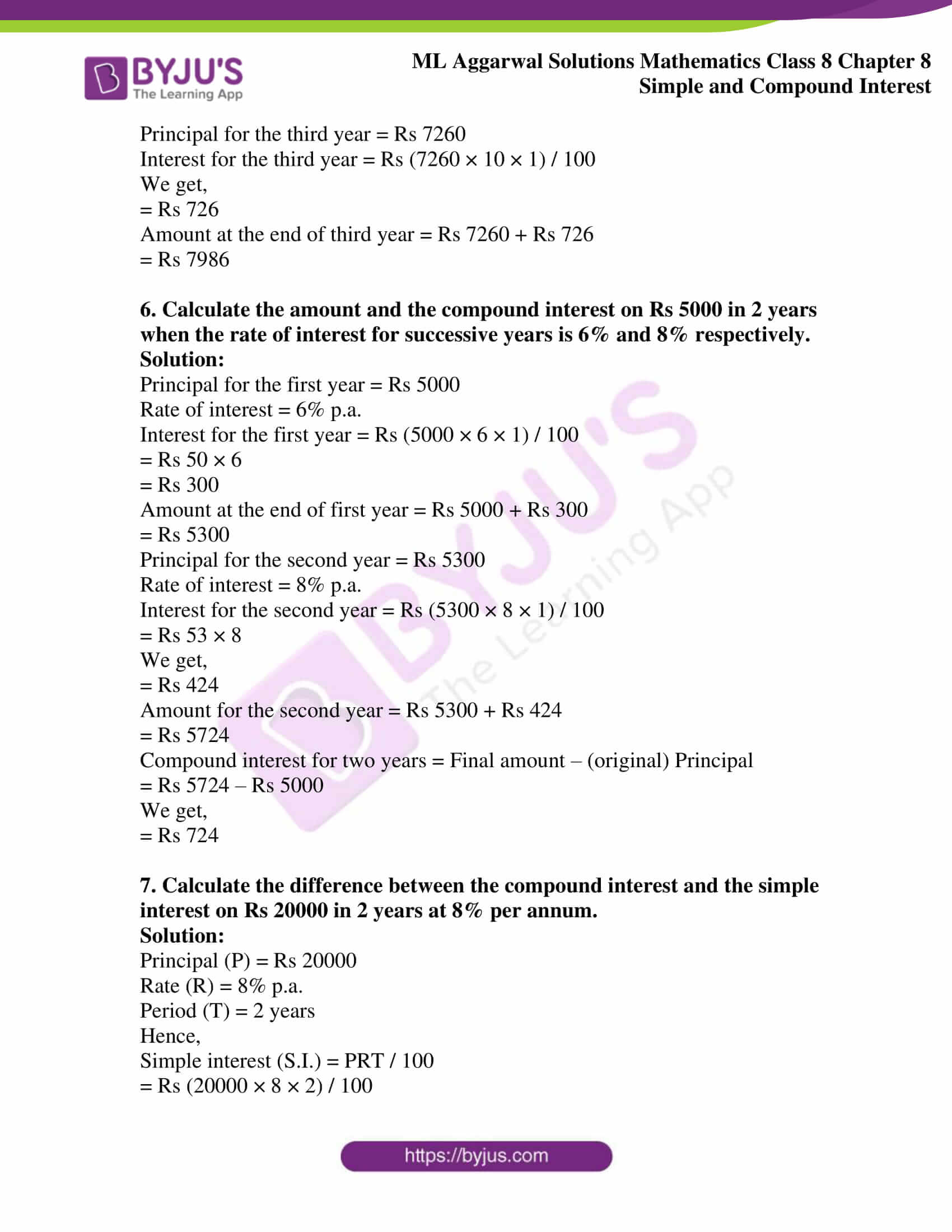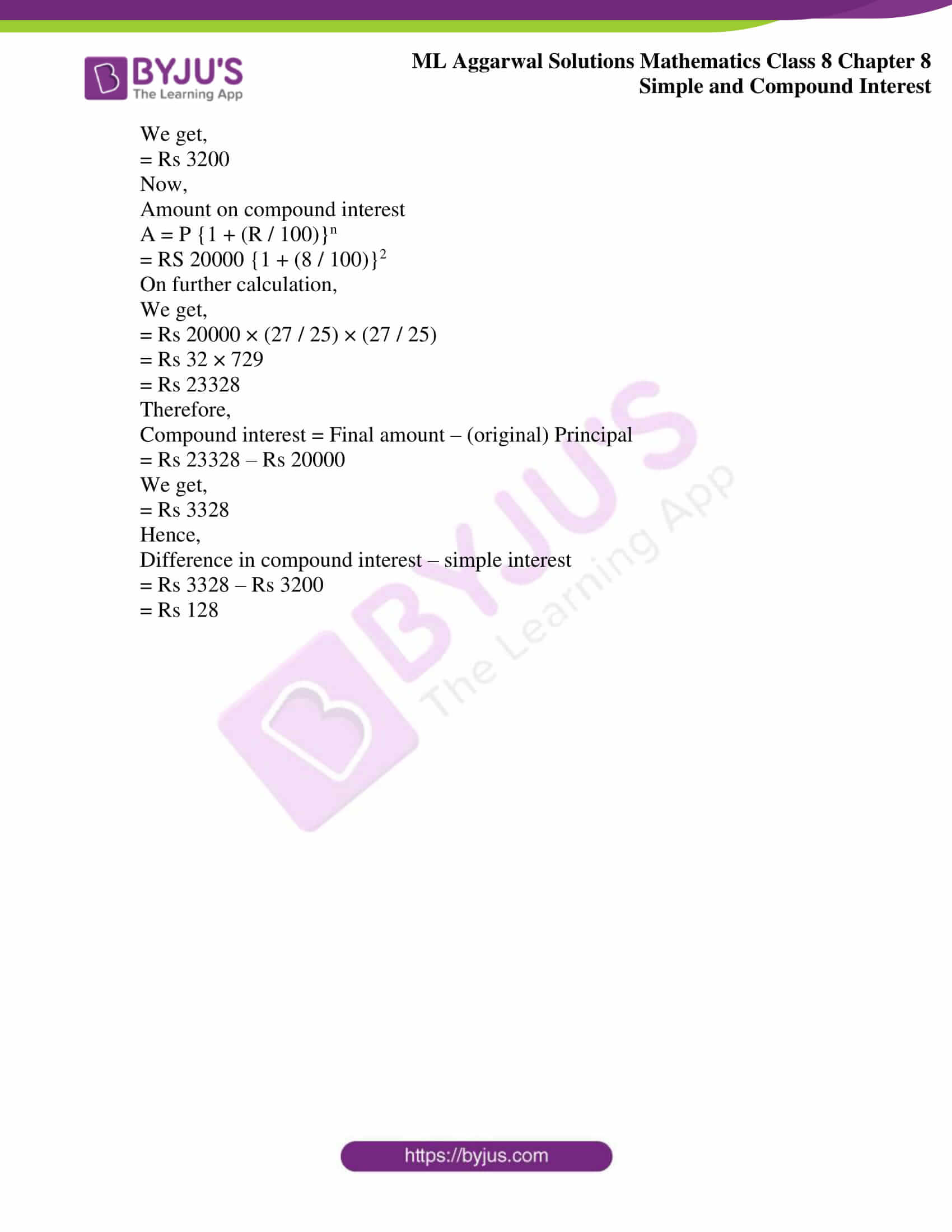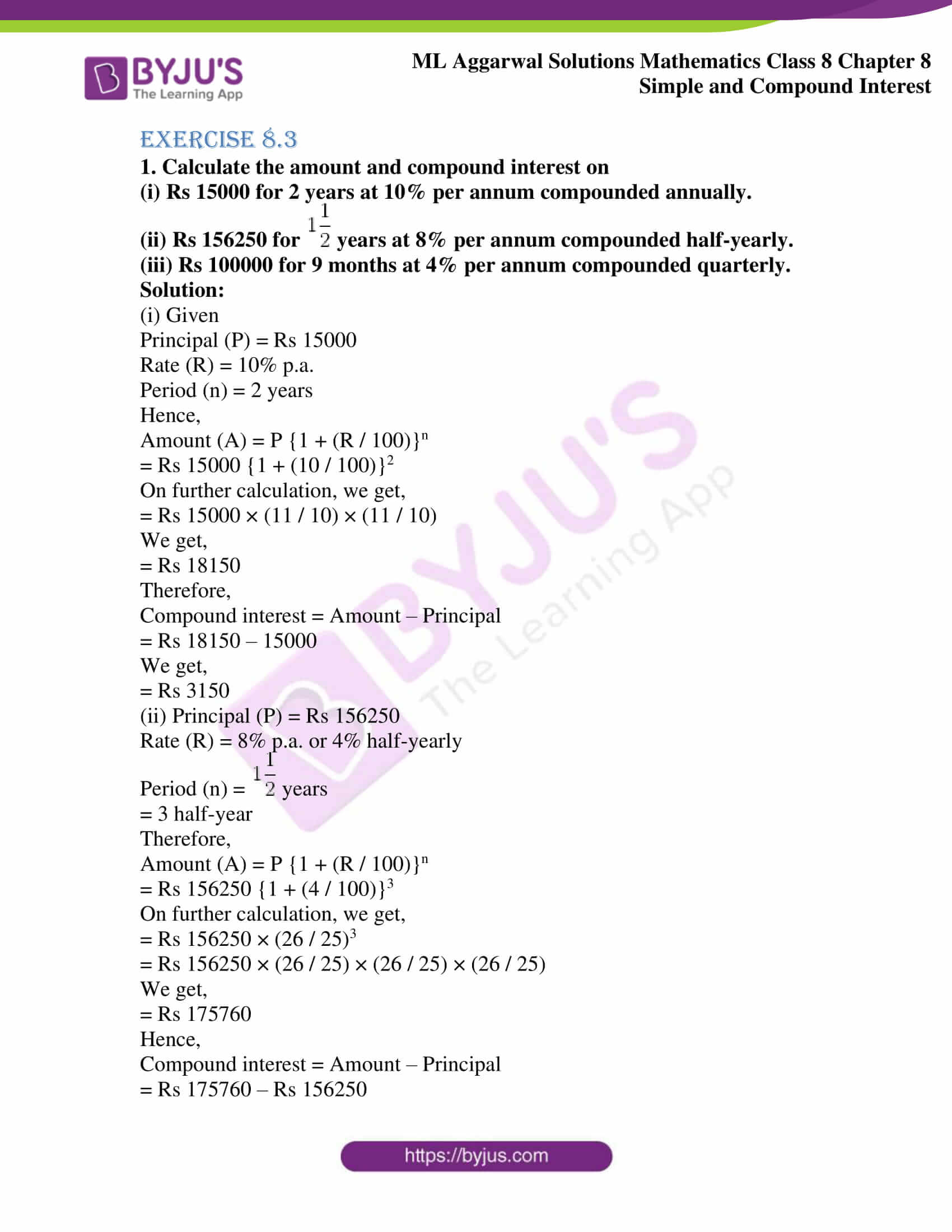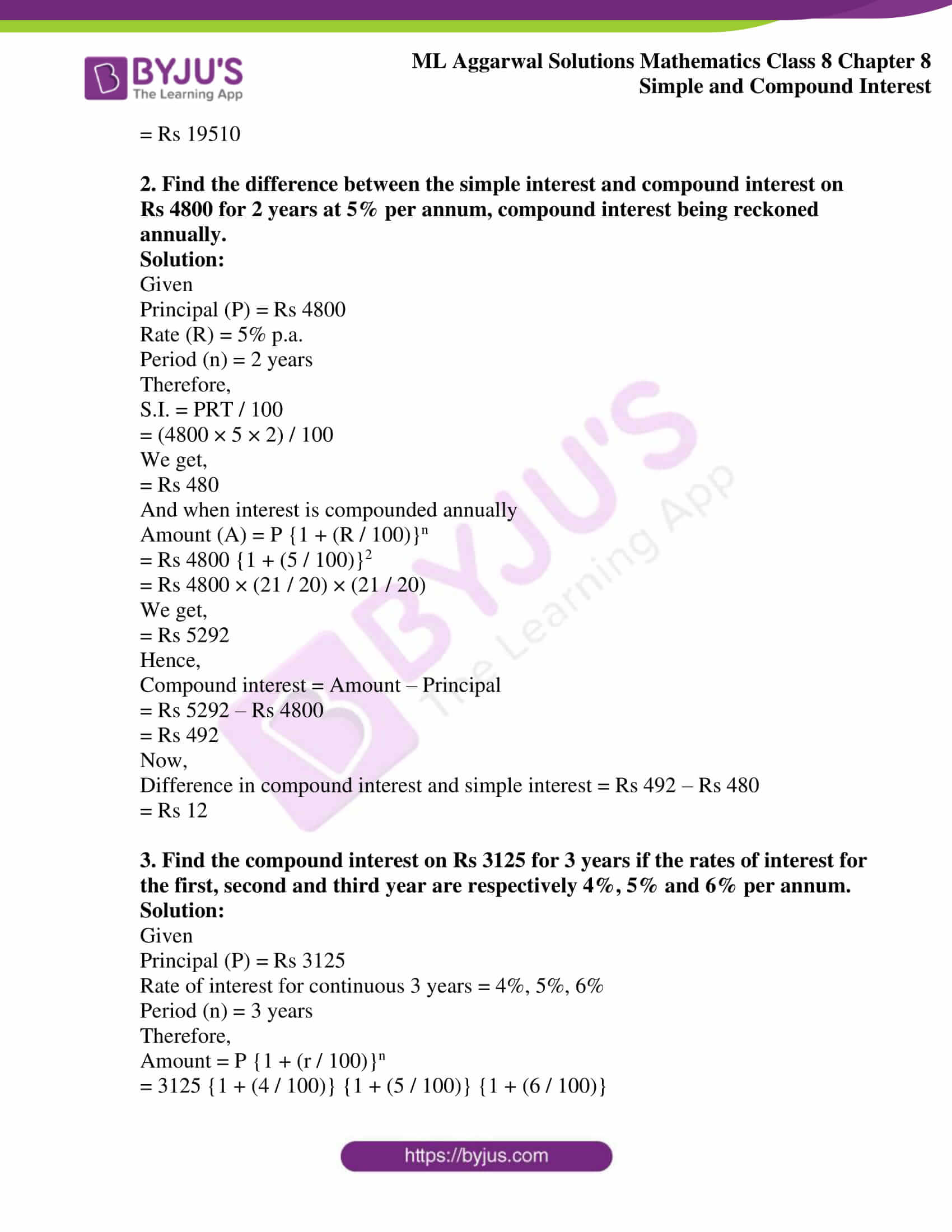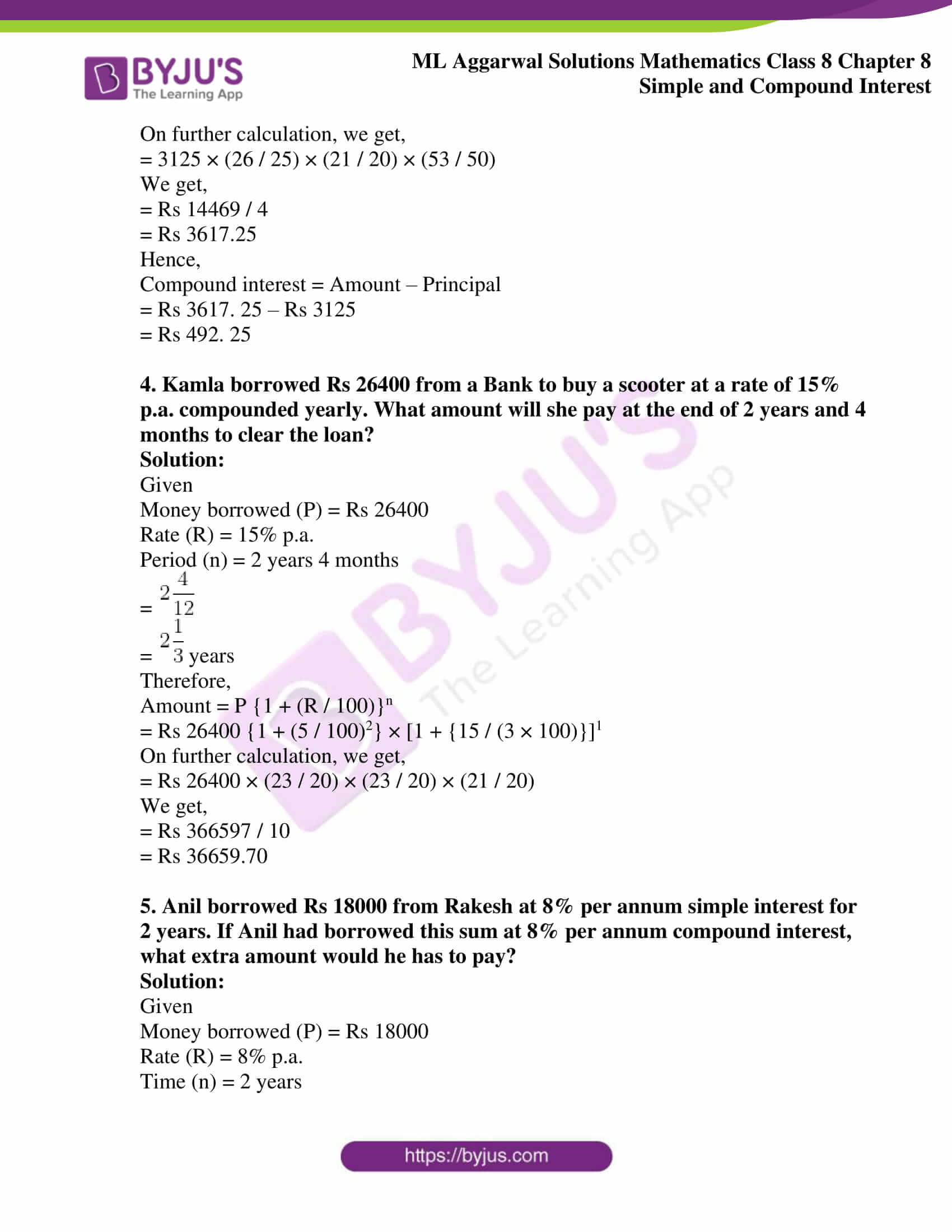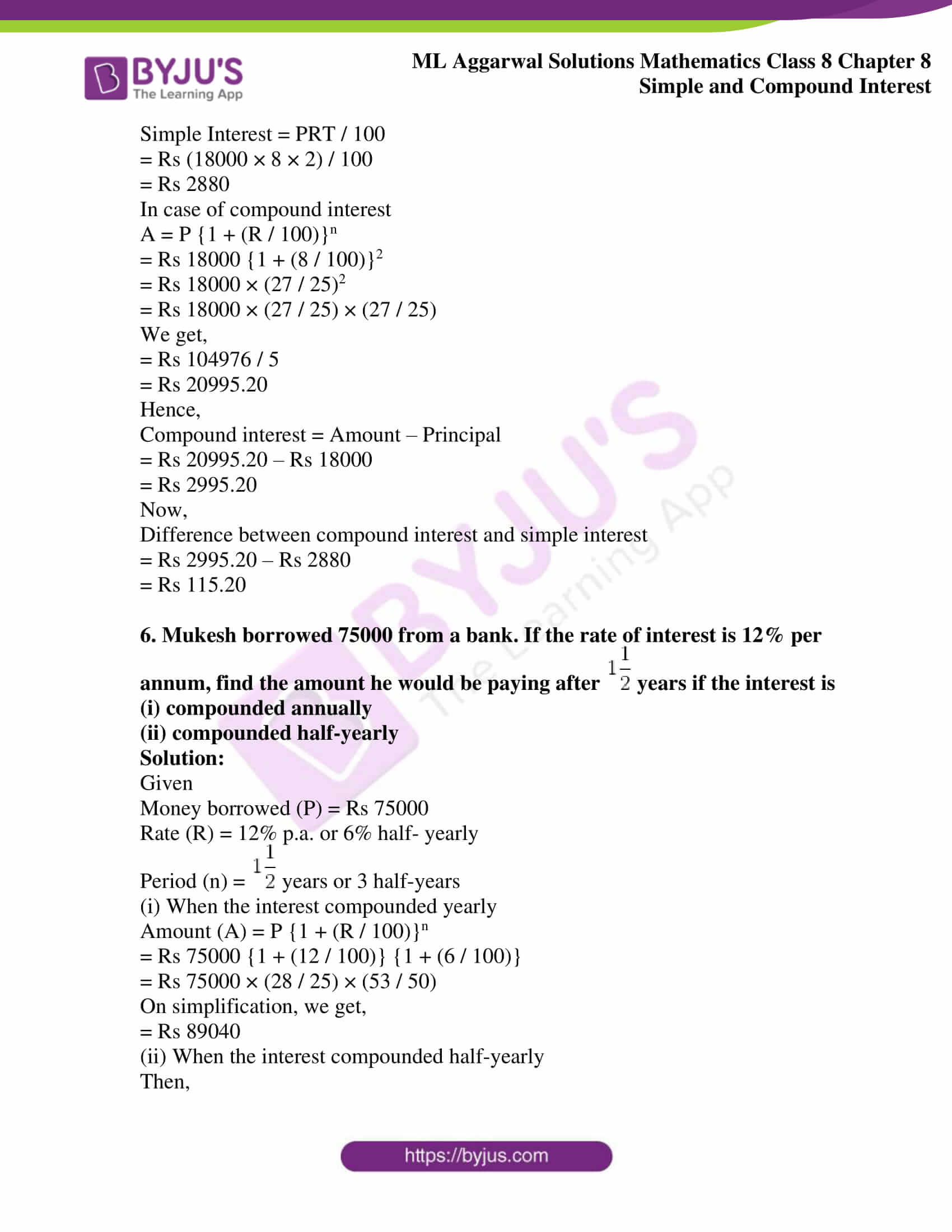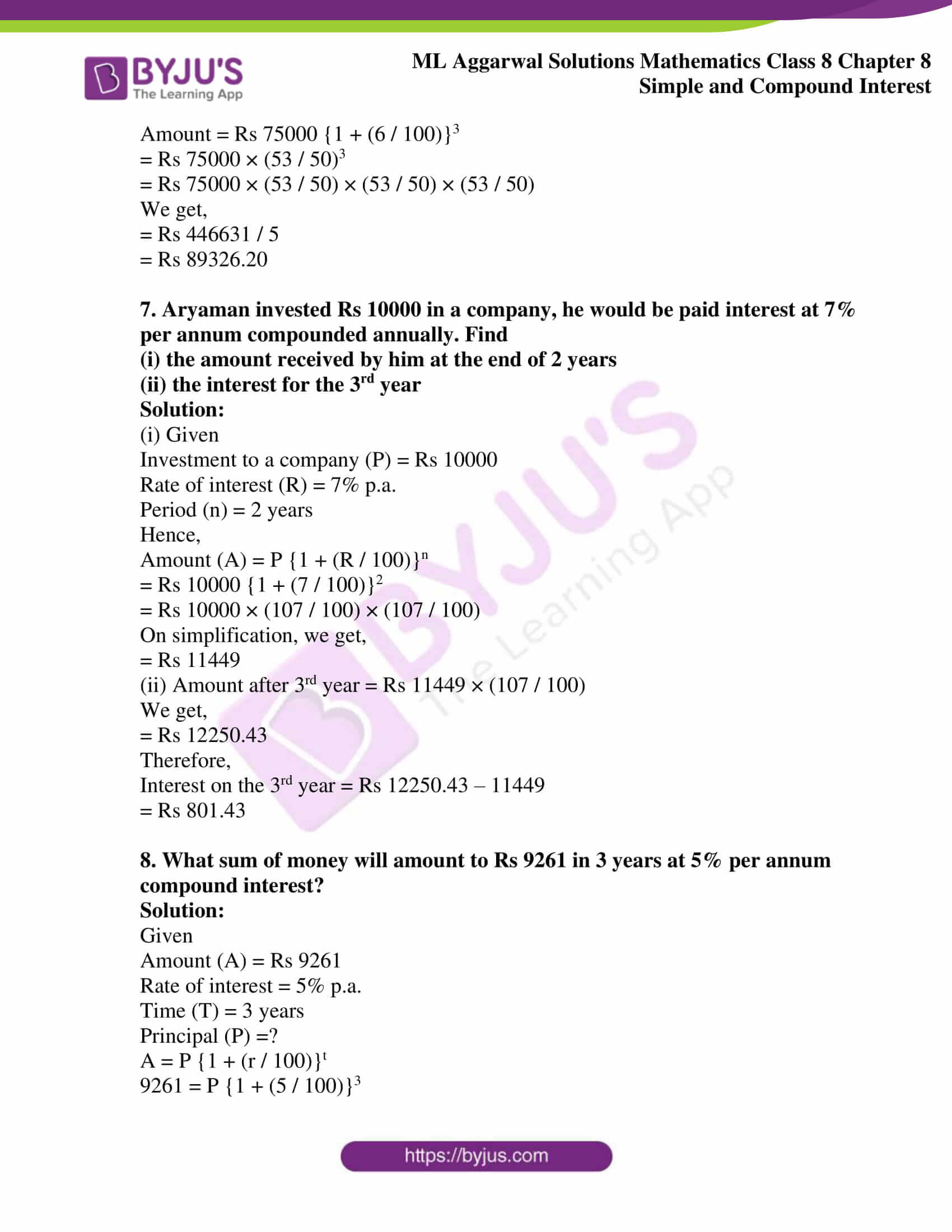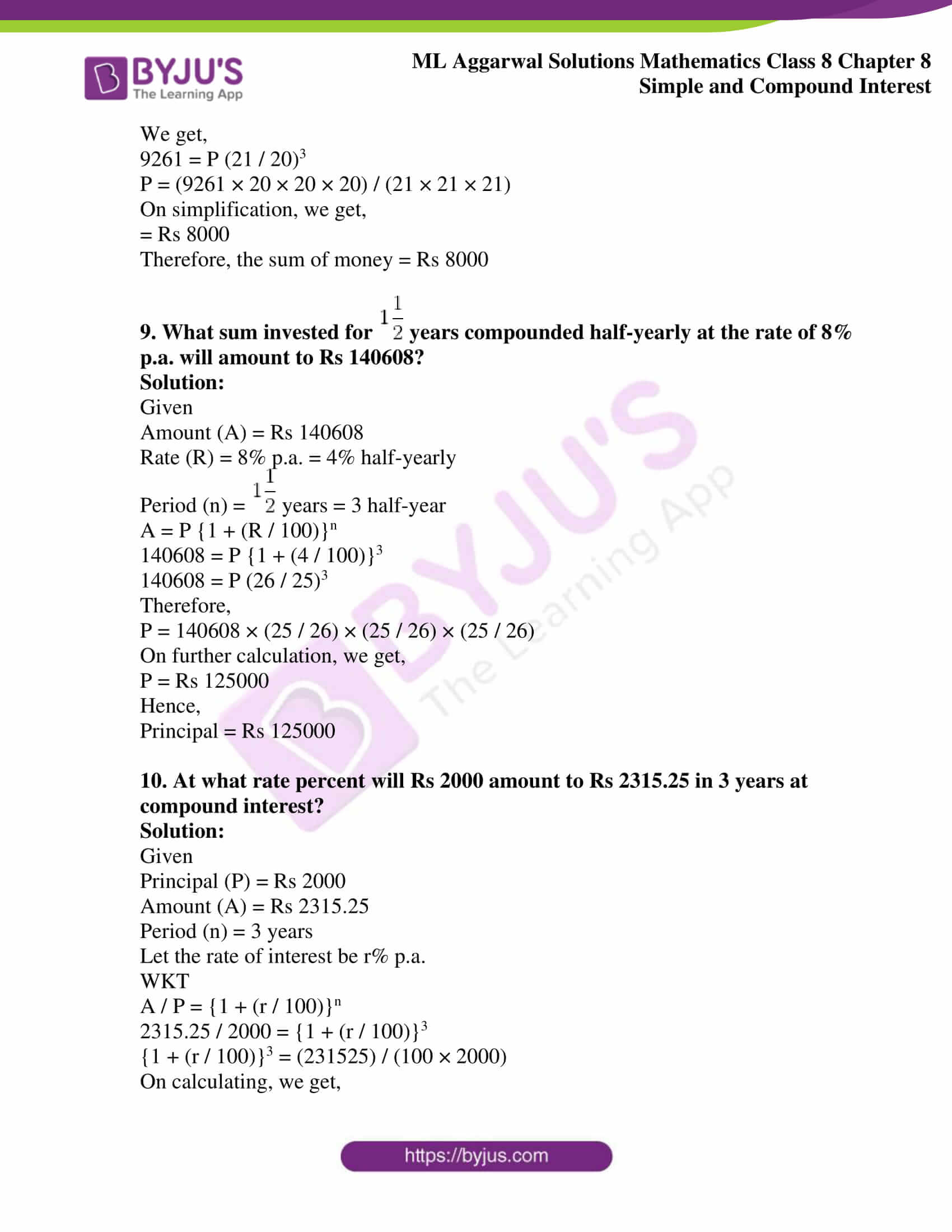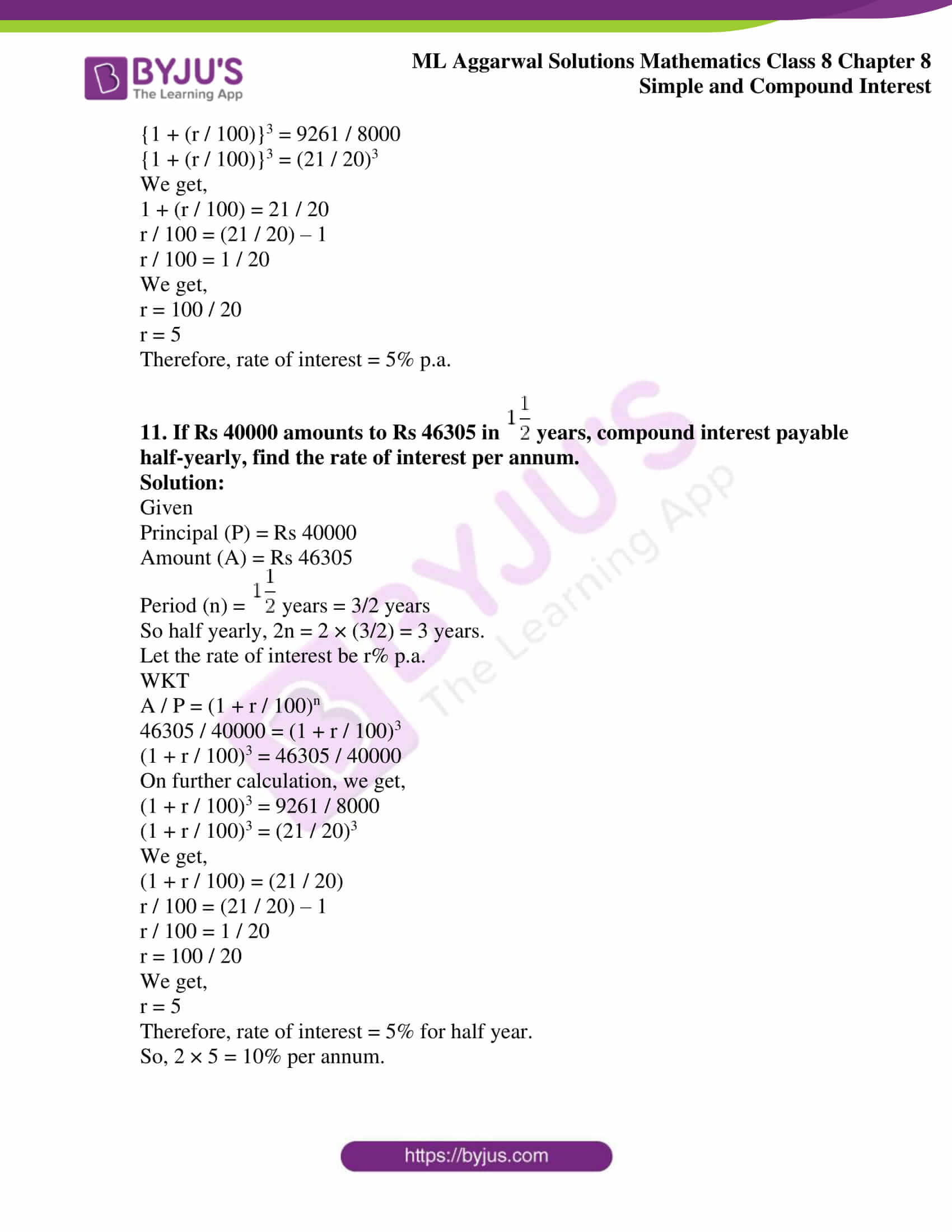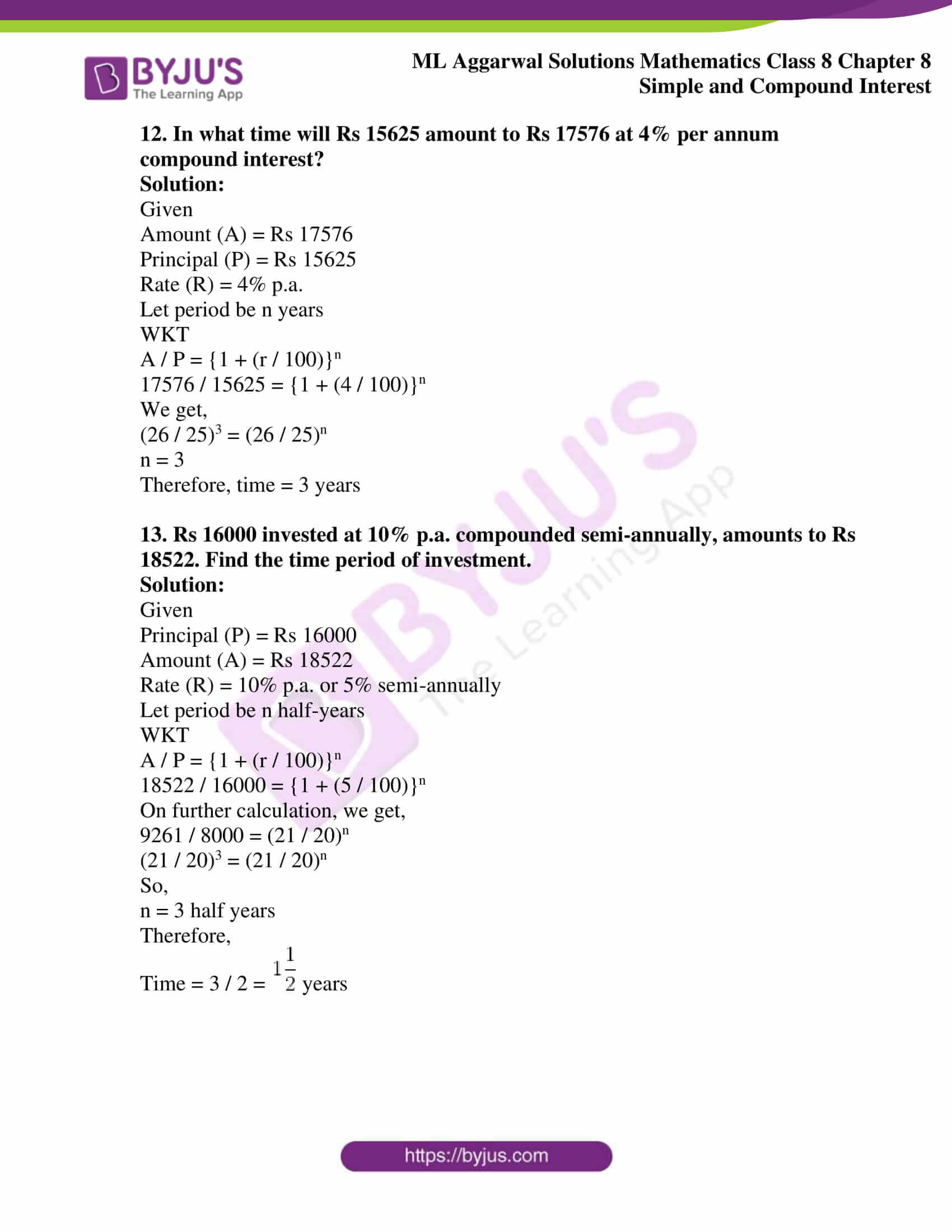## Access ML Aggarwal Solutions for Class 8 Maths Chapter 8 Simple and Compound Interest

Exercise 8.1

1. Find the simple interest on Rs 4000 at 7.5% p.a. for 3 years 3 months. Also, find the amount.

Solution:

Here

Principal (P) = Rs 4000

Rate of interest (R) = 7.5% p.a.

= (15 / 2) % p.a.

Time (T) = 3 years 3 months

=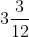years

=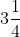years

= 13 / 4 years

Hence,

Simple Interest (I) = (P × R × T) / 100

= Rs {4000 × (15 / 2) × (13 / 4)} / 100

= Rs (4000 × 15 × 13) / (100 × 2 × 4)

On simplification, we get,

= Rs 5 × 15 × 13

= Rs 975

Therefore,

Amount = P + I

= Rs 4000 + Rs 975

= RS 4, 975

2. What sum of money will yield Rs 170.10 as simple interest in 2 years 3 months at 6% per annum?

Solution:

Here

I = Rs 170.10

T = 2 years 3 months

=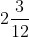years

=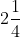years

= 9 / 4 years

R = 6%

Hence,

P = (I × 100) / (R × T)

= Rs (170.10 × 100) / {6 × (9 / 4)}

On calculating further, we get,

= Rs (170.10 × 100 × 4) / (6 × 9)

= Rs (17010 × 4) / (6 × 9)

= Rs (17010 × 2) / (3 × 9)

= Rs 34020 / 27

= Rs 1260

3. Find the rate of interest when Rs 800 fetches Rs 130 as a simple interest in 2 years 6 months.

Solution:

Here

P = Rs 800

T = 2 years 6 months

=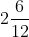years

=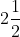years

= 5 / 2 years

Hence,

R = (I × 100) / (P × T)

= (130 × 100) / {800 × (5 / 2)} % p.a.

On simplification, we get,

= (130 × 100 × 2) / (800 × 5) % p.a.

= (130 × 2) / 40 %

= 130 / 20 % p.a.

= 13 / 2 %

= 6.5% p.a.

Therefore, the required rate of interest is 6.5% p.a.

4. Find the time when simple interest on Rs 3.3 lakhs at 6.5% per annum is Rs 75075.

Solution:

Here,

P = 3.3 lakhs

= Rs 3.3 × 100000

= Rs 330000

R = 6.5% per annum

I = Rs 75075

Hence,

T = (I × 100) / (P × R)

= (75075 × 100) / (330000 × 6.5) years

= (75075 × 100 × 10) / (330000 × 65) years

On further calculation, we get,

= (75075) / (330 × 65) years

= 1155 / 330 years

We get,

= 7 / 2 years

=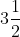years

5. Find the sum of money when

(i) simple interest at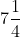% p.a. for years is Rs 2356.25

(ii) the final amount is Rs 11300 at 4% p.a. for 3 years 3 months.

Solution:

(i) Here,

I = Rs 2356.25

R =% p.a.

= 29 / 4 % p.a.

T =years

= 5 / 2 years

Hence,

P = (I × 100) / (R × T)

= Rs (2356.25 × 100) / (29 / 4) × (5 / 2)

On further calculation, we get,

= Rs (2356.25 × 100 × 4 × 2) / (29 × 5)

= Rs (235625 × 8) / (29 × 5)

We get,

= Rs (47125 × 8) / 29

= Rs 1625 × 8

= Rs 13000

(ii) Amount (A) = Rs 11300

Rate (R) = 4% p.a.

Time (T) = 3 years 3 months

=years

=years

= 13 / 4 years

Let the principal be Rs x

Hence,

S.I. = (P × R × T) / 100

= Rs (x × 4 × 13) / (100 × 4)

We get,

= Rs 13x / 100

Then,

Amount = Principal + Simple Interest

= Rs x + Rs 13x / 100

= Rs (x + 13x) / 100

We get,

= Rs (100x + 13x) / 100

= Rs (113x / 100)

But, the amount given is Rs 11300

Hence,

113x / 100 = 11300

x = 11300 × 100 / 113

x = 100 × 100

We get,

x = 10000

Therefore, principal (P) = Rs 10000

6. How long will it take a certain sum of money to triple itself at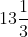% per annum simple interest?

Solution:

Let the sum of money be x

Amount = 3 × Rs x

= Rs 3x

Interest = Amount – Principal

= Rs 3x – Rs x

= Rs 2x

Rate =% p.a.

= 40 / 3 % p.a.

Time (T) = (I × 100) / (P × R)

= (2x × 100) / x × (40 / 3) years

On further calculation, we get,

= (2 × 100 × 3) / 40 years

= (100 × 3) / 20 years

We get,

= 5 × 3 years

= 15 years

7. At a certain rate of simple interest Rs 4050 amounts to Rs 4576.50 in 2 years. At the same rate of simple interest, how much would Rs 1 lakh amount to in 3 years?

Solution:

Here,

P = Rs 40000

A = Rs 4576.50

T = 2 years

Interest = Amount – Principal

= Rs 4576.50 – Rs 4050

= Rs 526.50

Let the rate of simple interest = R% per annum

Then,

R = (I × 100) / (P × T)

= (526.50 × 100) / (4050 × 2) % p.a.

On further calculation, we get,

= (526.50 × 10) / (405 × 2) % p.a.

= 5265 / 810 % p.a.

We get,

= 6.5% p.a.

Now,

P = Rs 1 lakh

= Rs 100000

R = 6.5% p.a.

T = 3 years

I = (P × R × T) / 100

= Rs (100000 × 6.5 × 3) / 100

We get,

= RS 1000 × 6.5 × 3

= Rs 19500

Amount = Principal + Interest

= Rs 100000 + Rs 19500

= Rs 119500

8. What sum of money invested at 7.5% p.a. simple interest for 2 years produces twice as much interest as Rs 9600 in 3 years 6 months at 10% p.a. simple interest?

Solution:

First Case:

Principal (P1) = Rs 9600

Rate (R1) = 10%

Period = (T) = 3 years 6 months

=years = 7 / 2 years

Simple interest = (P × R × T) / 100

= (9600 × 10 × 7) / (100 × 2)

We get,

= Rs 3360

Second case:

Simple interest = Rs 3360 × 2

= Rs 6720

Rate (R) = 7.5% p.a. and

Period (T) = 2 years

Therefore,

Principal = (S.I × 100) / (R × T)

= (6720 × 100) / (7.5 × 2)

= Rs (6720 × 100 × 10) / (75 × 2)

= 6720000 / 150

We get,

= Rs 44800

Exercise 8.2

1. Calculate the compound interest on Rs 6000 at 10% per annum for two years.

Solution:

Given

Rate of interest = 10% per annum

Principal for the first year = Rs 6000

Interest for the first year = Rs (6000 × 10 × 1) / 100

= Rs 600

Amount at the end of first year = Rs 6000 + Rs 600

= Rs 6600

Principal for the second year = Rs 6600

Interest for the second year = Rs (6600 × 10 × 1) / 100

= Rs 660

Amount for the second year = Rs 6600 + Rs 660

= Rs 7260

Therefore, compound interest for 2 years = final amount – (original) Principal

= Rs 7260 – Rs 6000

We get,

= Rs 1260

2. Salma borrowed from Mahila Samiti a sum of Rs 1875 to purchase a sewing machine. If the rate of interest is 4% per annum, what is the compound interest that she has to pay after 2 years?

Solution:

Principal for the first year = Rs 1875

Rate of interest = 4% p.a.

Interest for the first year = Rs (1875 × 4 × 1) / 100

= 75

Amount at the end of first year = Rs 1875 + Rs 75

= Rs 1950

Principal for the second year = Rs 1950

Interest for the second year = Rs (1950 × 4 × 1) / 100

= 78

Amount at the end of second year = Rs 1950 + Rs 78

= Rs 2028

Hence,

Compound interest paid by Salma = Final amount – (original) Principal

= Rs 2028 – Rs 1875

= Rs 153

3. Jacob invests Rs 12000 for 3 years at 10% per annum. Calculate the amount and the compound interest that Jacob will get after 3 years.

Solution:

Principal for the first year = Rs 12000

Rate of interest = 10% p.a.

Interest for the first year = Rs (12000 × 10 × 1) / 100

= Rs 1200

Amount at the end of first year = Rs 12000 + Rs 1200

= 13200

Principal for the second year = Rs 13200

Interest for the second year = Rs (13200 × 10 × 1) / 100

= Rs 1320

Amount at the end of second year = Rs 13200 + Rs 1320

= Rs 14520

Principal for the third year = Rs 14520

Interest for the third year = Rs (14520 × 10 × 1) / 100

= Rs 1452

Amount at the end of third year = Rs 14520 + Rs 1452

= Rs 15972

Hence,

Compound interest for 3 year = Final amount – (original) Principal

= Rs 15972 – Rs 12000

= Rs 3972

4. A man invests Rs 46875 at 4% per annum compound interest for 3 years.

Calculate:

(i) the interest for the first year

(ii) the amount standing to his credit at the end of second year

(iii) the interest for the third year

Solution:

(i) Principal for the first year = Rs 46875

Rate of interest = 4% per annum

Therefore,

Interest for the first year = Rs (46875 × 4 × 1) / 100

We get,

= Rs 46875 / 25

= Rs 1875

Hence, interest for the first year is Rs 1875

(ii) Amount at the end of first year

= Rs 46875 + Rs 1875

We get,

= Rs 48750

Principal for the second year = Rs 48750

Interest for the second year = Rs (48750 × 4 × 1) / 100

= Rs 48750 / 25

We get,

= Rs 1950

Amount at the end of second year = Rs 48750 + Rs 1950

We get,

= Rs 50700

Hence, the amount at the end of second year is Rs 50700

(iii) Principal for the third year = Rs 50700

Interest for the third year = Rs (50700 × 4 × 1) / 100

We get,

= Rs 507 × 4

= Rs 2028

Hence, the interest for the third year is Rs 2028

5. Calculate the compound interest for the second year on Rs 6000 invested for 3 years at 10% p.a. Also find the sum due at the end of third year.

Solution:

Principal for the first year = Rs 6000

Rate of interest = 10% p.a.

Interest for the first year = Rs (6000 × 10 × 1) / 100

= Rs 600

Amount at the end of first year = Rs 6000 + Rs 600

= Rs 6600

Principal for the second year = Rs 6600

Interest for the second year = Rs (6600 × 10 × 1) / 100

We get,

= Rs 660

Amount at the end of second year = Rs 6600 + Rs 660

= Rs 7260

Compound interest for the second year = Final amount – (original) Principal

= Rs 7260 – Rs 6000

= Rs 1260

Principal for the third year = Rs 7260

Interest for the third year = Rs (7260 × 10 × 1) / 100

We get,

= Rs 726

Amount at the end of third year = Rs 7260 + Rs 726

= Rs 7986

6. Calculate the amount and the compound interest on Rs 5000 in 2 years when the rate of interest for successive years is 6% and 8% respectively.

Solution:

Principal for the first year = Rs 5000

Rate of interest = 6% p.a.

Interest for the first year = Rs (5000 × 6 × 1) / 100

= Rs 50 × 6

= Rs 300

Amount at the end of first year = Rs 5000 + Rs 300

= Rs 5300

Principal for the second year = Rs 5300

Rate of interest = 8% p.a.

Interest for the second year = Rs (5300 × 8 × 1) / 100

= Rs 53 × 8

We get,

= Rs 424

Amount for the second year = Rs 5300 + Rs 424

= Rs 5724

Compound interest for two years = Final amount – (original) Principal

= Rs 5724 – Rs 5000

We get,

= Rs 724

7. Calculate the difference between the compound interest and the simple interest on Rs 20000 in 2 years at 8% per annum.

Solution:

Principal (P) = Rs 20000

Rate (R) = 8% p.a.

Period (T) = 2 years

Hence,

Simple interest (S.I.) = PRT / 100

= Rs (20000 × 8 × 2) / 100

We get,

= Rs 3200

Now,

Amount on compound interest

A = P {1 + (R / 100)}n

= RS 20000 {1 + (8 / 100)}2

On further calculation,

We get,

= Rs 20000 × (27 / 25) × (27 / 25)

= Rs 32 × 729

= Rs 23328

Therefore,

Compound interest = Final amount – (original) Principal

= Rs 23328 – Rs 20000

We get,

= Rs 3328

Hence,

Difference in compound interest – simple interest

= Rs 3328 – Rs 3200

= Rs 128

Exercise 8.3

1. Calculate the amount and compound interest on

(i) Rs 15000 for 2 years at 10% per annum compounded annually.

(ii) Rs 156250 for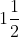years at 8% per annum compounded half-yearly.

(iii) Rs 100000 for 9 months at 4% per annum compounded quarterly.

Solution:

(i) Given

Principal (P) = Rs 15000

Rate (R) = 10% p.a.

Period (n) = 2 years

Hence,

Amount (A) = P {1 + (R / 100)}n

= Rs 15000 {1 + (10 / 100)}2

On further calculation, we get,

= Rs 15000 × (11 / 10) × (11 / 10)

We get,

= Rs 18150

Therefore,

Compound interest = Amount – Principal

= Rs 18150 – 15000

We get,

= Rs 3150

(ii) Principal (P) = Rs 156250

Rate (R) = 8% p.a. or 4% half-yearly

Period (n) =years

= 3 half-year

Therefore,

Amount (A) = P {1 + (R / 100)}n

= Rs 156250 {1 + (4 / 100)}3

On further calculation, we get,

= Rs 156250 × (26 / 25)3

= Rs 156250 × (26 / 25) × (26 / 25) × (26 / 25)

We get,

= Rs 175760

Hence,

Compound interest = Amount – Principal

= Rs 175760 – Rs 156250

= Rs 19510

2. Find the difference between the simple interest and compound interest on Rs 4800 for 2 years at 5% per annum, compound interest being reckoned annually.

Solution:

Given

Principal (P) = Rs 4800

Rate (R) = 5% p.a.

Period (n) = 2 years

Therefore,

S.I. = PRT / 100

= (4800 × 5 × 2) / 100

We get,

= Rs 480

And when interest is compounded annually

Amount (A) = P {1 + (R / 100)}n

= Rs 4800 {1 + (5 / 100)}2

= Rs 4800 × (21 / 20) × (21 / 20)

We get,

= Rs 5292

Hence,

Compound interest = Amount – Principal

= Rs 5292 – Rs 4800

= Rs 492

Now,

Difference in compound interest and simple interest = Rs 492 – Rs 480

= Rs 12

3. Find the compound interest on Rs 3125 for 3 years if the rates of interest for the first, second and third year are respectively 4%, 5% and 6% per annum.

Solution:

Given

Principal (P) = Rs 3125

Rate of interest for continuous 3 years = 4%, 5%, 6%

Period (n) = 3 years

Therefore,

Amount = P {1 + (r / 100)}n

= 3125 {1 + (4 / 100)} {1 + (5 / 100)} {1 + (6 / 100)}

On further calculation, we get,

= 3125 × (26 / 25) × (21 / 20) × (53 / 50)

We get,

= Rs 14469 / 4

= Rs 3617.25

Hence,

Compound interest = Amount – Principal

= Rs 3617. 25 – Rs 3125

= Rs 492. 25

4. Kamla borrowed Rs 26400 from a Bank to buy a scooter at a rate of 15% p.a. compounded yearly. What amount will she pay at the end of 2 years and 4 months to clear the loan?

Solution:

Given

Money borrowed (P) = Rs 26400

Rate (R) = 15% p.a.

Period (n) = 2 years 4 months

=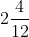=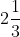years

Therefore,

Amount = P {1 + (R / 100)}n

= Rs 26400 {1 + (5 / 100)2} × [1 + {15 / (3 × 100)}]1

On further calculation, we get,

= Rs 26400 × (23 / 20) × (23 / 20) × (21 / 20)

We get,

= Rs 366597 / 10

= Rs 36659.70

5. Anil borrowed Rs 18000 from Rakesh at 8% per annum simple interest for 2 years. If Anil had borrowed this sum at 8% per annum compound interest, what extra amount would he has to pay?

Solution:

Given

Money borrowed (P) = Rs 18000

Rate (R) = 8% p.a.

Time (n) = 2 years

Simple Interest = PRT / 100

= Rs (18000 × 8 × 2) / 100

= Rs 2880

In case of compound interest

A = P {1 + (R / 100)}n

= Rs 18000 {1 + (8 / 100)}2

= Rs 18000 × (27 / 25)2

= Rs 18000 × (27 / 25) × (27 / 25)

We get,

= Rs 104976 / 5

= Rs 20995.20

Hence,

Compound interest = Amount – Principal

= Rs 20995.20 – Rs 18000

= Rs 2995.20

Now,

Difference between compound interest and simple interest

= Rs 2995.20 – Rs 2880

= Rs 115.20

6. Mukesh borrowed 75000 from a bank. If the rate of interest is 12% per annum, find the amount he would be paying afteryears if the interest is

(i) compounded annually

(ii) compounded half-yearly

Solution:

Given

Money borrowed (P) = Rs 75000

Rate (R) = 12% p.a. or 6% half- yearly

Period (n) =years or 3 half-years

(i) When the interest compounded yearly

Amount (A) = P {1 + (R / 100)}n

= Rs 75000 {1 + (12 / 100)} {1 + (6 / 100)}

= Rs 75000 × (28 / 25) × (53 / 50)

On simplification, we get,

= Rs 89040

(ii) When the interest compounded half-yearly

Then,

Amount = Rs 75000 {1 + (6 / 100)}3

= Rs 75000 × (53 / 50)3

= Rs 75000 × (53 / 50) × (53 / 50) × (53 / 50)

We get,

= Rs 446631 / 5

= Rs 89326.20

7. Aryaman invested Rs 10000 in a company, he would be paid interest at 7% per annum compounded annually. Find

(i) the amount received by him at the end of 2 years

(ii) the interest for the 3rd year

Solution:

(i) Given

Investment to a company (P) = Rs 10000

Rate of interest (R) = 7% p.a.

Period (n) = 2 years

Hence,

Amount (A) = P {1 + (R / 100)}n

= Rs 10000 {1 + (7 / 100)}2

= Rs 10000 × (107 / 100) × (107 / 100)

On simplification, we get,

= Rs 11449

(ii) Amount after 3rd year = Rs 11449 × (107 / 100)

We get,

= Rs 12250.43

Therefore,

Interest on the 3rd year = Rs 12250.43 – 11449

= Rs 801.43

8. What sum of money will amount to Rs 9261 in 3 years at 5% per annum compound interest?

Solution:

Given

Amount (A) = Rs 9261

Rate of interest = 5% p.a.

Time (T) = 3 years

Principal (P) =?

A = P {1 + (r / 100)}t

9261 = P {1 + (5 / 100)}3

We get,

9261 = P (21 / 20)3

P = (9261 × 20 × 20 × 20) / (21 × 21 × 21)

On simplification, we get,

= Rs 8000

Therefore, the sum of money = Rs 8000

9. What sum invested foryears compounded half-yearly at the rate of 8% p.a. will amount to Rs 140608?

Solution:

Given

Amount (A) = Rs 140608

Rate (R) = 8% p.a. = 4% half-yearly

Period (n) =years = 3 half-year

A = P {1 + (R / 100)}n

140608 = P {1 + (4 / 100)}3

140608 = P (26 / 25)3

Therefore,

P = 140608 × (25 / 26) × (25 / 26) × (25 / 26)

On further calculation, we get,

P = Rs 125000

Hence,

Principal = Rs 125000

10. At what rate percent will Rs 2000 amount to Rs 2315.25 in 3 years at compound interest?

Solution:

Given

Principal (P) = Rs 2000

Amount (A) = Rs 2315.25

Period (n) = 3 years

Let the rate of interest be r% p.a.

WKT

A / P = {1 + (r / 100)}n

2315.25 / 2000 = {1 + (r / 100)}3

{1 + (r / 100)}3 = (231525) / (100 × 2000)

On calculating, we get,

{1 + (r / 100)}3 = 9261 / 8000

{1 + (r / 100)}3 = (21 / 20)3

We get,

1 + (r / 100) = 21 / 20

r / 100 = (21 / 20) – 1

r / 100 = 1 / 20

We get,

r = 100 / 20

r = 5

Therefore, rate of interest = 5% p.a.

11. If Rs 40000 amounts to Rs 46305 inyears, compound interest payable half-yearly, find the rate of interest per annum.

Solution:

Given

Principal (P) = Rs 40000

Amount (A) = Rs 46305

Period (n) =years = 3/2 years

So half yearly, 2n = 2 × (3/2) = 3 years.

Let the rate of interest be r% p.a.

WKT

A / P = (1 + r / 100)n

46305 / 40000 = (1 + r / 100)3

(1 + r / 100)3 = 46305 / 40000

On further calculation, we get,

(1 + r / 100)3 = 9261 / 8000

(1 + r / 100)3 = (21 / 20)3

We get,

(1 + r / 100) = (21 / 20)

r / 100 = (21 / 20) – 1

r / 100 = 1 / 20

r = 100 / 20

We get,

r = 5

Therefore, rate of interest = 5% for half year.

So, 2 × 5 = 10% per annum.

12. In what time will Rs 15625 amount to Rs 17576 at 4% per annum compound interest?

Solution:

Given

Amount (A) = Rs 17576

Principal (P) = Rs 15625

Rate (R) = 4% p.a.

Let period be n years

WKT

A / P = {1 + (r / 100)}n

17576 / 15625 = {1 + (4 / 100)}n

We get,

(26 / 25)3 = (26 / 25)n

n = 3

Therefore, time = 3 years

13. Rs 16000 invested at 10% p.a. compounded semi-annually, amounts to Rs 18522. Find the time period of investment.

Solution:

Given

Principal (P) = Rs 16000

Amount (A) = Rs 18522

Rate (R) = 10% p.a. or 5% semi-annually

Let period be n half-years

WKT

A / P = {1 + (r / 100)}n

18522 / 16000 = {1 + (5 / 100)}n

On further calculation, we get,

9261 / 8000 = (21 / 20)n

(21 / 20)3 = (21 / 20)n

So,

n = 3 half years

Therefore,

Time = 3 / 2 =years